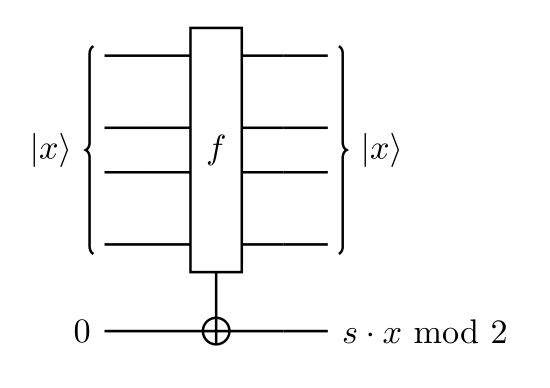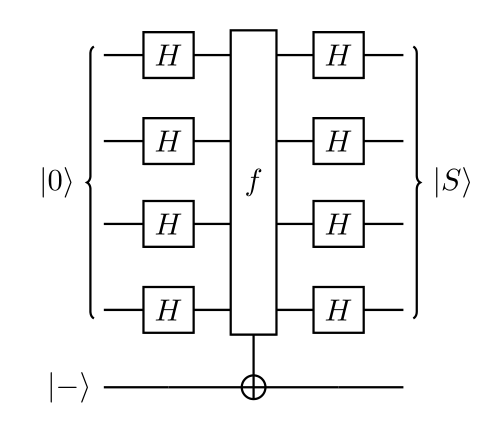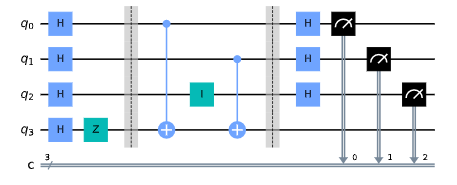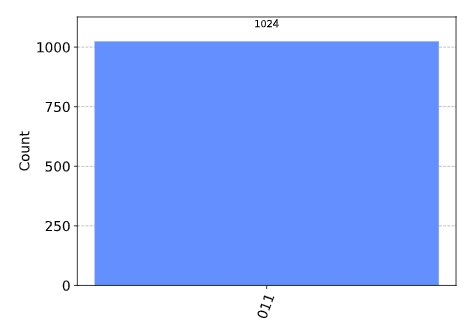# Bernstein-Vazirani算法

[toc]

## 1. Bernstein-Vazirani算法

### 1.1 Bernstein-Vazirani问题

f(\{x_0,x_1,x_2,...\}) \rightarrow 0 \textrm{ or } 1 \textrm{ where } x_n \textrm{ is }0 \textrm{ or } 1### 1.2 经典解决方案

f_s(x) = s \cdot x \mod 2

Input(x)
100…0
010…0
001…0
000…1

### 1.3 量子解决方案

1. 将输入量子位初始化为 |0\rangle^{\otimes n} 状态，输出量子位为 |{-}\rangle
2. 对输入寄存器应用哈达玛门
3. 查询oracle
4. 对输入寄存器应用哈达玛门
5. 测量|a\rangle \xrightarrow{H^{\otimes n}} \frac{1}{\sqrt{2^n}} \sum_{x\in \{0,1\}^n} (-1)^{a\cdot x}|x\rangle.

H|0\rangle = \tfrac{1}{\sqrt{2}}(|0\rangle + |1\rangle) \\\\ H|1\rangle = \tfrac{1}{\sqrt{2}}(|0\rangle - |1\rangle)

H|a\rangle = \frac{1}{\sqrt{2}}\sum_{x\in \{0,1\}} (-1)^{a\cdot x}|x\rangle.

H^{\otimes 2}|00\rangle = \tfrac{1}{2}(|00\rangle + |01\rangle + |10\rangle + |11\rangle) \\\\ H^{\otimes 2}|01\rangle = \tfrac{1}{2}(|00\rangle - |01\rangle + |10\rangle - |11\rangle)\\\\ H^{\otimes 2}|10\rangle = \tfrac{1}{2}(|00\rangle + |01\rangle - |10\rangle - |11\rangle)\\\\ H^{\otimes 2}|11\rangle = \tfrac{1}{2}(|00\rangle - |01\rangle - |10\rangle + |11\rangle)

H^{\otimes 2}|a\rangle = \frac{1}{2}\sum_{x\in \{0,1\}^2} (-1)^{a\cdot x}|x\rangle

|00\dots 0\rangle \xrightarrow{H^{\otimes n}} \frac{1}{\sqrt{2^n}} \sum_{x\in \{0,1\}^n} |x\rangle

|x \rangle \xrightarrow{f_s} (-1)^{s\cdot x} |x \rangle

|00\dots 0\rangle \xrightarrow{H^{\otimes n}} \frac{1}{\sqrt{2^n}} \sum_{x\in \{0,1\}^n} |x\rangle \xrightarrow{f_a} \frac{1}{\sqrt{2^n}} \sum_{x\in \{0,1\}^n} (-1)^{a\cdot x}|x\rangle

\frac{1}{\sqrt{2^n}} \sum_{x\in \{0,1\}^n} (-1)^{a\cdot x}|x\rangle \xrightarrow{H^{\otimes n}} |a\rangle

## 2.样例

1. 两个量子比特的寄存器初始化为零:
\lvert \psi_0 \rangle = \lvert 0 0 \rangle
1. 对两个量子比特应用哈达玛门：
\lvert \psi_1 \rangle = \frac{1}{2} \left( \lvert 0 0 \rangle + \lvert 0 1 \rangle + \lvert 1 0 \rangle + \lvert 1 1 \rangle \right)
1. 对于字符串 s=11 ，量子oracle执行以下操作:
|x \rangle \xrightarrow{f_s} (-1)^{x\cdot 11} |x \rangle.\\\\ \lvert \psi_2 \rangle = \frac{1}{2} \left( (-1)^{00\cdot 11}|00\rangle + (-1)^{01\cdot 11}|01\rangle + (-1)^{10\cdot 11}|10\rangle + (-1)^{11\cdot 11}|11\rangle \right)\\\\ \lvert \psi_2 \rangle = \frac{1}{2} \left( \lvert 0 0 \rangle - \lvert 0 1 \rangle - \lvert 1 0 \rangle + \lvert 1 1 \rangle \right)
1. 对两个量子比特应用哈达玛门：
\lvert \psi_3 \rangle = \lvert 1 1 \rangle
1. 测量以找到秘密字符串 s=11

from qiskit_textbook.widgets import bv_widget
bv_widget(2, "11")


## 3.Qiskit实现

# initialization
import matplotlib.pyplot as plt
import numpy as np

# importing Qiskit
from qiskit import IBMQ, Aer
from qiskit.providers.ibmq import least_busy
from qiskit import QuantumCircuit, ClassicalRegister, QuantumRegister, transpile, assemble

# import basic plot tools
from qiskit.visualization import plot_histogram


n = 3 # number of qubits used to represent s
s = '011'   # the hidden binary string


# We need a circuit with n qubits, plus one auxiliary qubit
# Also need n classical bits to write the output to
bv_circuit = QuantumCircuit(n+1, n)

# put auxiliary in state |->
bv_circuit.h(n)
bv_circuit.z(n)

# Apply Hadamard gates before querying the oracle
for i in range(n):
bv_circuit.h(i)

# Apply barrier
bv_circuit.barrier()

# Apply the inner-product oracle
s = s[::-1] # reverse s to fit qiskit's qubit ordering
for q in range(n):
if s[q] == '0':
bv_circuit.i(q)
else:
bv_circuit.cx(q, n)

# Apply barrier
bv_circuit.barrier()

#Apply Hadamard gates after querying the oracle
for i in range(n):
bv_circuit.h(i)

# Measurement
for i in range(n):
bv_circuit.measure(i, i)

bv_circuit.draw()### 3a.使用模拟器进行实验

# use local simulator
aer_sim = Aer.get_backend('aer_simulator')
shots = 1024
qobj = assemble(bv_circuit)
results = aer_sim.run(qobj).result()### 3b.使用真实设备进行实验

# Load our saved IBMQ accounts and get the least busy backend device with less than or equal to 5 qubits
provider = IBMQ.get_provider(hub='ibm-q')
provider.backends()
backend = least_busy(provider.backends(filters=lambda x: x.configuration().n_qubits <= 5 and
x.configuration().n_qubits >= 2 and
not x.configuration().simulator and x.status().operational==True))
print("least busy backend: ", backend)


# Run our circuit on the least busy backend. Monitor the execution of the job in the queue
from qiskit.tools.monitor import job_monitor

shots = 1024
transpiled_bv_circuit = transpile(bv_circuit, backend)
job = backend.run(transpiled_bv_circuit, shots=shots)

job_monitor(job, interval=2)


# Get the results from the computation
results = job.result()



## 4.练习

1. 使用下面的小部件来查看Bernstein-Vazirani算法在不同oracle上的运行情况:
from qiskit_textbook.widgets import bv_widget
bv_widget(3, "011", hide_oracle=False)

1. 上面Bernstein-Vazirani的实现是为了一个秘密的位字符串 s=011 。修改秘密字符串 s=011 的实现。结果和你预期的一样吗?解释一下。
2. 上面Bernstein-Vazirani的实现是为了一个秘密的位字符串 s=011 。修改秘密字符串 s = 11101101 的实现。结果和你预期的一样吗?解释一下。

## 5.参考文献

1.Ethan Bernstein and Umesh Vazirani (1997) “Quantum Complexity Theory” SIAM Journal on Computing, Vol. 26, No. 5: 1411-1473, doi:10.1137/S0097539796300921.

2.Jiangfeng Du, Mingjun Shi, Jihui Wu, Xianyi Zhou, Yangmei Fan, BangJiao Ye, Rongdian Han (2001) “Implementation of a quantum algorithm to solve the Bernstein-Vazirani parity problem without entanglement on an ensemble quantum computer”, Phys. Rev. A 64, 042306, 10.1103/PhysRevA.64.042306, arXiv:quant-ph/0012114.

import qiskit.tools.jupyter
%qiskit_version_table
`